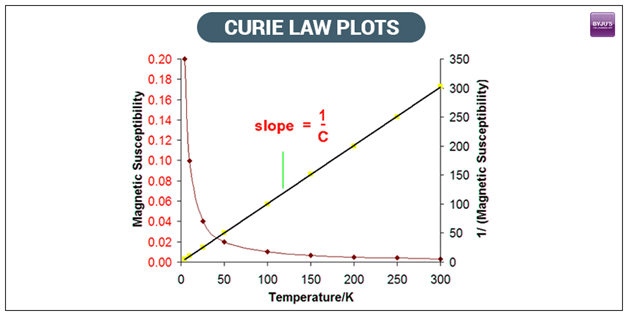# Curie's Law - Statement and Formula

We have discussed various important laws of Physics in previous sessions. In this article, let us learn in detail the curie’s law, curie’s law formula, paramagnetism and curie temperature.

## Curie’s Law

According to Curie’s Law, the magnetization in a paramagnetic material is directly proportional to the applied magnetic field. If the object is heated, the magnetization is viewed to be inversely proportional to the temperature. The law was discovered by the French physicist, Pierre Curie.
Curie’s Law Formula
Curie’s Law can be framed into an equation.
M = C x (B/T)
Wherein,
M = Magnetism
B = Magnetic field(in Tesla)
T = absolute temperature (in Kelvins)
C = Curie constant
Curie’s law holds good for high temperature and not so strong magnetic fields.## What Is Meant By Paramagnetic?

Paramagnetism relates to materials such as platinum or aluminium that are magnetized in a magnetic field and their magnetism becomes extinct when the field doesn’t exist. Ferromagnetism is the materials like nickel and iron that retain their magnetic properties when the field is erased.

## What Is The Curie Temperature?

The Curie temperature is the one at which ferromagnetic material turns to paramagnetic on heating. This kind of transition is used in optical storage media for erasing and inserting new data.

## Do you know what a compass is and why do the needles in a compass move the way they move? Watch the video to find answers to these questions!## Frequently Asked Questions – FAQs

Q1

### State Curie’s law?

According to Curie’s Law, the magnetization in a paramagnetic material is directly proportional to the applied magnetic field.
Q2

### Give the Curie’s law formula

The Curie’s law formula is given by:
M = C x (B/T)
Wherein,
M = Magnetism
B = Magnetic field(in Tesla)
T = absolute temperature (in Kelvins)
C = Curie constant
Q3

### State true or false: Curie’s law does not hold good for high temperatures.

FALSE. Curie’s law holds good for high temperatures.
Q4

### Nickel is ferromagnetic or paramagnetic material?

Nickel is a ferromagnetic material.
Q5

### Define Curie temperature.

It is the temperature at which a ferromagnetic material turns to paramagnetic on heating.

Visit “BYJU’S – The Learning App” to know more about Curie constant table along with types of magnetic materials with their properties.

Test Your Knowledge On Curies Law!